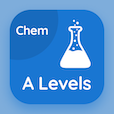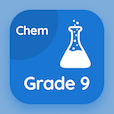A Level Courses

A Level Chemistry Practice Tests

A Level Chemistry Tests

The Book Introduction to Lattice Energy Multiple Choice Questions (MCQ Quiz) PDF, Introduction to Lattice Energy MCQs, download to learn online a level chemistry courses. Study Lattice Energy Multiple Choice Questions and Answers (MCQs), Introduction to Lattice Energy quiz answers PDF for two year degree programs. The eBook Introduction to Lattice Energy MCQ App Download: lattice energy value, ion polarization test prep for colleges that offer online classes.

The MCQ: The lattice energy is always PDF, "Introduction to Lattice Energy MCQ" App Download (Free) with endothermic, exothermic, ectothermic, and hypothermic choices for two year degree programs. Practice introduction to lattice energy quiz questions, download Amazon eBook (Free Sample) for online colleges for teaching.

## Chemistry: Introduction to Lattice Energy MCQs

MCQ: The lattice energy is always

A) endothermic
B) exothermic
C) ectothermic
D) hypothermic

MCQ: When ions of opposite charges come together to form a crystal lattice is called

A) bond energy
B) crystal energy
C) lattice energy
D) none of above

MCQ: Under standard conditions the energy with enthalpy change when 1 mole of an ionic compound is formed from its gaseous ions is

A) bond energy
B) crystal energy
C) lattice energy
D) structural energy

### Practice Tests: A Level Chemistry Exam Prep

Download A level Chemistry Quiz App, College Chemistry MCQ App, and 9th Grade Chemistry MCQs App to install for Android & iOS devices. These Apps include complete analytics of real time attempts with interactive assessments. Download Play Store & App Store Apps & Enjoy 100% functionality with subscriptions!